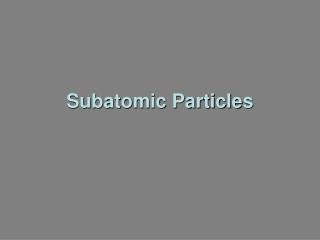DownloadDownload PresentationSubatomic Particles

# Subatomic Particles

Download Presentation## Subatomic Particles

- - - - - - - - - - - - - - - - - - - - - - - - - - - E N D - - - - - - - - - - - - - - - - - - - - - - - - - - -
##### Presentation Transcript

1. Subatomic Particles

2. Calculating Average Atomic Mass • expressed in atomic mass units (amu) • done because actual masses so small and hard to work with • based on carbon-12 assigned mass of exactly 12 amu • weighted average of all naturally occurring isotopes.

3. change %abundances to decimals for each isotope Multiply abundance by atomic mass of isotope Add products to get average atomic mass for element for 75.77% = 0.7577 for 24.33% = 0.2433 Example Chlorine has two isotopes. The isotope with a mass of 34.969 amu has a relative abundance of 75.77%. The isotope with a mass of 36.966 amu has a relative abundance of24.33%. Calculate the atomic mass of chlorine. x 34.969 = 26.49 amu x 36.966 = 8.994 amu for Cl atomic mass = 35.484 = 35.48 amu